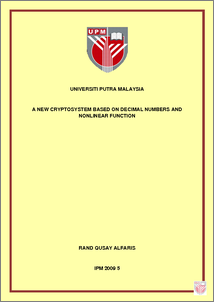# A New Cryptosystem Based on Decimal Numbers and Nonlinear Function

## Citation

Alfaris, Rand Qusay (2009) A New Cryptosystem Based on Decimal Numbers and Nonlinear Function. PhD thesis, Universiti Putra Malaysia.

## Abstract

We introduce a new cryptosystem, with new digital signature procedure. This cryptosystem is based on decimal numbers and nonlinear function. Unlike other cryptosystems, this system does not depend on prime or integer numbers and it does not depend on group structure, ¯nite ¯eld or discrete logarithmic equation. The idea is about using decimal numbers and thus the only integer number will be the plaintext. A secret key is shared between Alice and Bob to construct their decimal public keys, so that, we can say this cryptosystem is symmetric and asym- metric. We choose to distribute the secret key through the classic Di±e-Hellman protocol after introducing some modi¯cation on it; this modi¯cation depends on the extension of the theory of the modulus to be applicable on the real numbers. We prove there is no loss of any bit of information when we use the decimal num- bers during the encryption and the decryption by introducing the rounding o® concept as a function. This function plays the primary role in proving a new the- orem called \Rounding theorem". A new theory for the security is established, where basically, it depends on the properties of the decimal numbers and the cu- mulative and truncation errors that will occur during the attack, because every attack requires solving a system of non-linear equations. The decimal cryptosys- tem does not depend on large numbers because it deals with numbers between zero and one. Therefore, this cryptosystem can be considered faster than the known cryptosystems.Preview
PDF
IPM_2009_5a.pdfView Item